# Triangle Inequality Theorem

Triangle Inequality Theorem

Let's say there are three random spots $$A$$, $$B$$, and $$C$$ on a floor and join the paths $$AB$$, $$BC$$, and $$AC$$.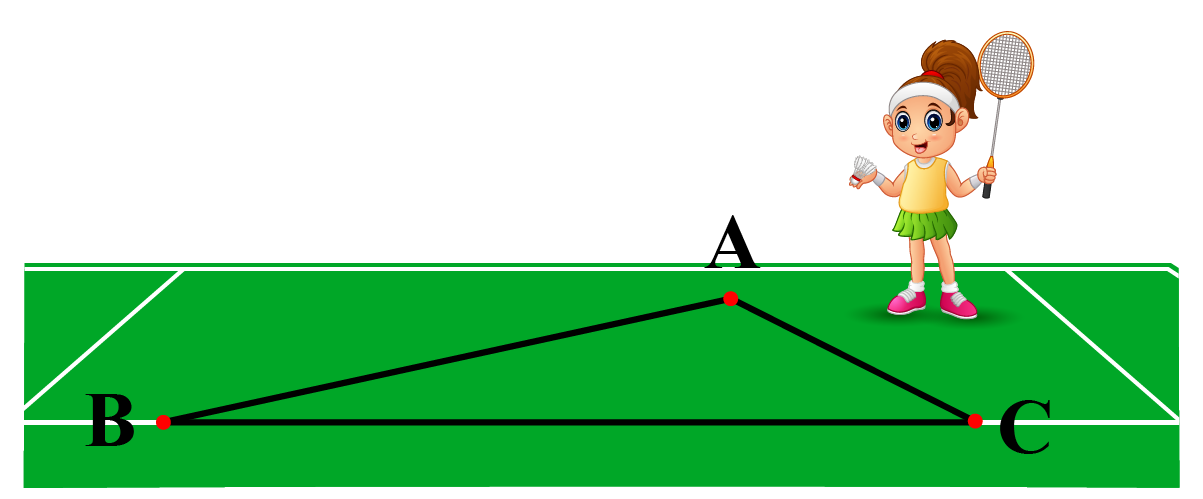We then ask Saumya to start from $$A$$ and reach $$C$$, walking along these three paths.

She can first walk along $$\overline{AB}$$ and then walk along $$\overline{BC}$$ to reach $$C$$ or she can directly walk along $$\overline{AC}$$.

What do you think? What path will she prefer?

She will naturally prefer the direct path $$\overline{AC}$$ because $$\overline{AB}+\overline{BC}>\overline{AC}$$.

Certainly, most of us will follow that path only.

$$\overline{AC}$$ because $$\overline{AB}+\overline{BC}>\overline{AC}$$, gives us the crux of the Triangle Inequality Theorem, i.e, “the sum of any two sides of a triangle is greater than its third side."

In this lesson, we will explore triangle inequality theorem, triangle inequality theorem-proof, triangle inequality theorem problems and try our hands-on triangle inequality theorem calculator and interactive problems to have a better understanding of it.

Let's begin!

## Lesson Plan

 1 What Is Triangle Inequality Theorem? 2 Solved Examples on Triangle Inequality Theorem 3 Tips and Tricks 4 Important Notes on Triangle Inequality Theorem 5 Interactive Questions on Triangle Inequality Theorem

## What Is Triangle Inequality Theorem?

The Triangle inequality theorem states, "The sum of any two sides of a triangle is greater than its third side."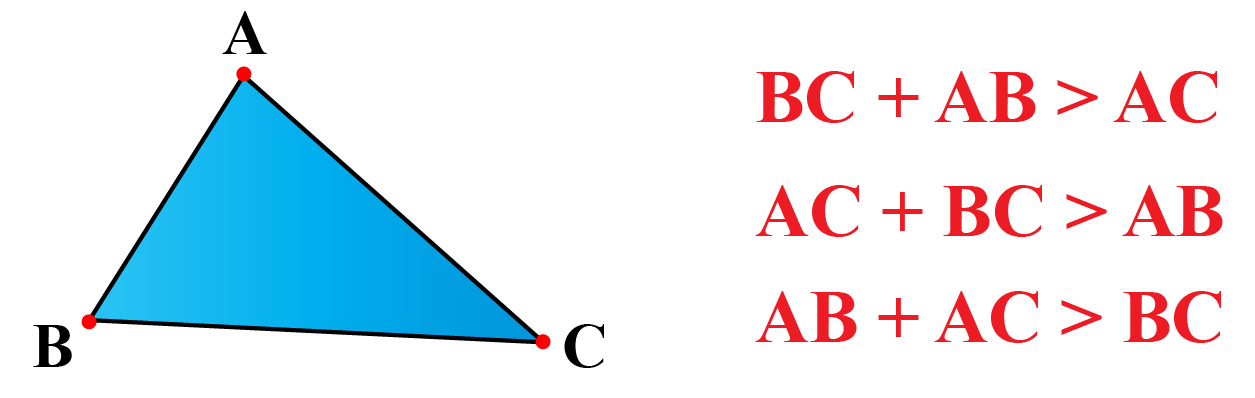Let's do an activity to implement this theorem, and later we will solve some triangle inequality theorem problems.

Take a few small strips of different lengths, say, 2 cm, 3 cm, 4 cm, 5 cm, ...,10 cm.

Form a triangle taking any three strips.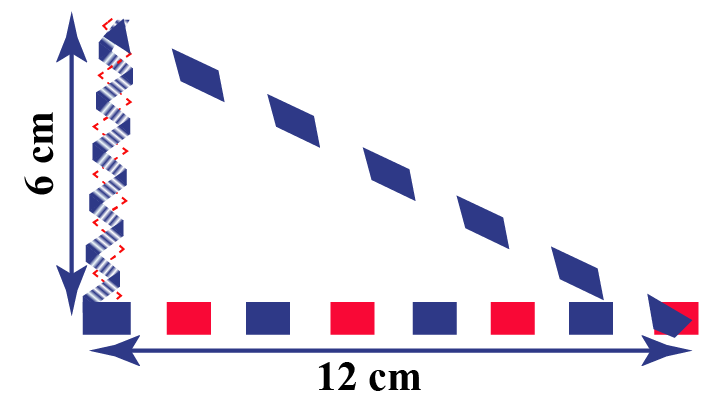Suppose you first choose two strips of length 6 cm and 12 cm.

What do you think about the length of the third strip?

Your third strip has to be of length more than 12 – 6 = 6 cm, and less than 12 + 6 = 18 cm.

Repeat this activity by choosing different combinations of the three strips.

Do you know what we observe when we do this?

We observe that the sum of the lengths of any two sides of a triangle is greater than the third side.Tips and Tricks
1. The sum of the lengths of any two sides of a triangle is greater than the length of the third side.
2. The difference between the lengths of any two lengths is less than the length of the third side.

## What is the General Formula of Triangle Inequality Theorem?

### Triangle Inequality Theorem Proof

Consider a triangle $$ABC$$ as shown below.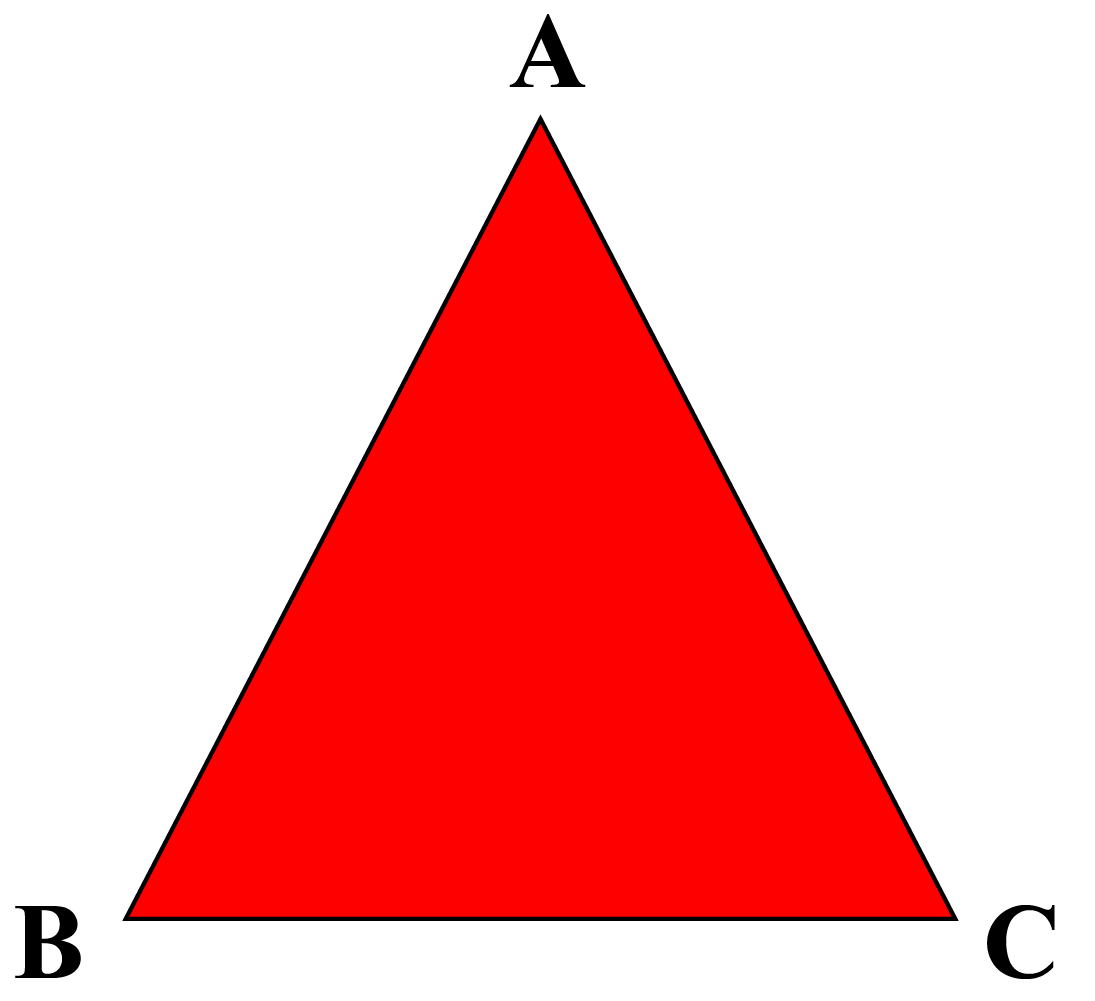We will do construction in this triangle.

Let's produce the side $$BA$$ to a point $$D$$ such that $$AC=AD$$.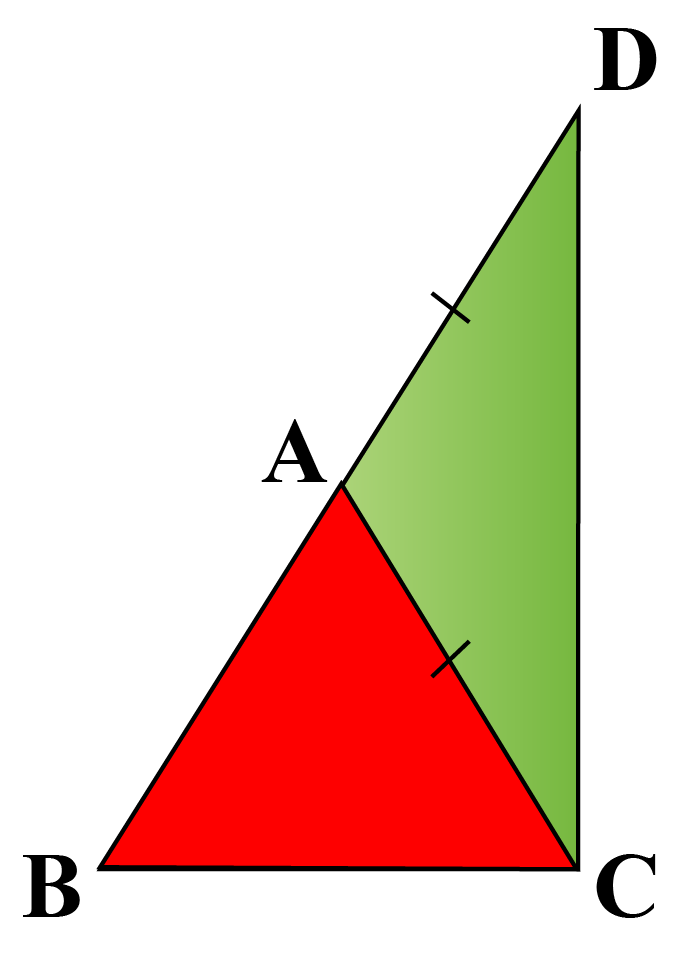Applying Angle Sum Property in $$\triangle BDC$$, we get:

\begin{align}\angle BDC+\angle CBD+\angle BCD&=180^{\circ}\\\angle BDC+\angle CBD+90^{\circ}&=180^{\circ}\\\angle BDC+\angle CBD&=90^{\circ}\end{align}

So, $$\angle BCD > \angle BDC$$.

Since the side opposite to the greater angle is longer, we have $$BD>BC$$.

This implies:

\begin{align}BD&>BC\\AB+AD&>BC\\AB+AC&>BC\end{align}

Hence proved.

A triangle inequality theorem calculator is designed as well to discover the multiple possibilities of the triangle formation.

More Important Topics
Numbers
Algebra
Geometry
Measurement
Money
Data
Trigonometry
Calculus